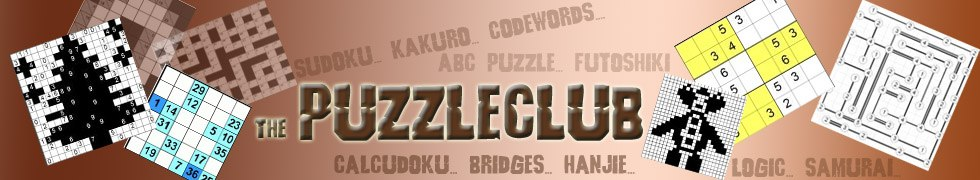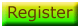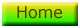# Killer Sudoku Tips

We've seen two major techniques you can use to solve a killer sudoku puzzle. Here is another strategy that you should be aware of and can be used to good effect to help solve a killer sudoku puzzle.

## 4 - The rule of 21 or 45

Depending on the size of a puzzle, you know that it must contain some combination of 1 - 6 or 1 - 9 in each row, column, 3 x 2 or 3 x 3. Therefore you know that the sum of each of those regions must be 21 or 45 respectively. This is because 1+2+3+4+5+6 = 21 and 1+2+3+4+5+6+7+8+9 = 45.

Let's return to an example of a 3 x 2 puzzle to see how we can use this sum rule, here the rule of 21, to help us place numbers and solve our puzzle. Here are the top two rows of a puzzle: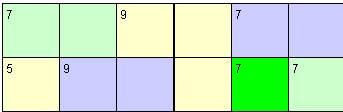Now, we know that the top row of this puzzle must sum to 21. And therefore we know that the two cells that make up the '9' on the top row must sum to '7', because the other four cells on the row must sum to 14 and 21 - 14 is 7. Now, because we know that these two cells sum to '7', and that the 9 is composed of three cells, we know for certain that the cell on the second row must contain a '2'. Thus we place it on our grid: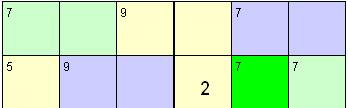Be sure to bear this rule in mind whilst solving puzzles, as it can be quite a powerful tactic to help you place numbers on the grid. Used in combination with the rules outlined on the previous page and a little practice, you will stand yourself in excellent stead to solve killer sudoku puzzles!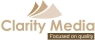©   |     |